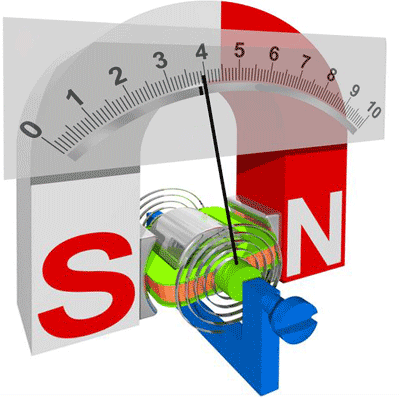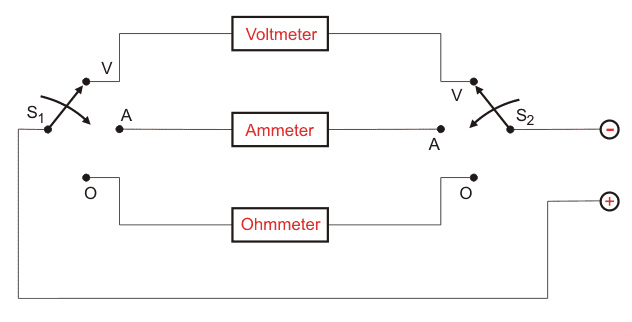# Analog Multimeter

Multimeters as the name suggest the meters that we use to measure multiple quantities with the same instrument. The most basic multimeter measures voltage, current, and resistance. Since we use it for measuring current (A), voltage (V) and resistance (Ohm), we call it as AVO meter. We can categorise the multimeters into two groups, namely analog multimeter and digital multimeter. We will discuss here in this article about analog multimeter.

Analog multimeter was first of its type, but due to latest technological development after development of digital multimeters, nowadays it is of less use. However, despite such advancements, it is still essential, and we can’t neglect it. An analog multimeter is a PMMC meter. It works based on the d’Arsonval galvanometer principle. It consists a needle to indicate the measured value on the scale. A coil moves in a magnetic field when current passes through it. The indicating needle is fastened to the coil. During the flow of current through the coil, a deflecting torque gets produced due to which the coil rotates at some angle, and the pointer moves over a graduated scale. A pair of hairsprings is attached to the moving spindle to provide the controlling torque. In a multimeter, the galvanometer is a left- zero-type instrument, i.e. needle rests to the extreme left of the scale from where the scale begins with zero.The meter acts as an ammeter with a low series resistance to measure direct current. For measuring high current, we connect a shunt resistor across the galvanometer so that the current through the galvanometer does not cross its maximum allowed value. Here, a significant portion of the current to be measured bypasses through the shunt. With that shunt resistance, an analog multimeter can measure even milli-ammeter or ammeter ranges of current.

For DC voltage measurement, the primary instrument becomes a DC voltage measuring apparatus or DC voltmeter. By adding a multiplier resistance, an analog multimeter can measure the voltage from milli-volts to kilovolts, and this meter works as a millivoltmeter, a voltmeter or even as a kilo voltmeter.

By adding a battery and a resistance network, this instrument can work as an ohmmeter. We can change the range of the ohmmeter by connecting a switch to a suitable shunt resistance. By selecting different values of shunt resistance, we can obtain different scales of resistance measurement. Here below we are showing a basic block diagram of an analog multimeter.Here we are using two switches namely S1 and S2 to select the desired meter. We may use additional range-selector switches to choose particular range required in reading amperes, volts, and ohms. We use a rectifier to measure an AC voltage or current with the multimeter.

• A sudden change in signal can detect by analog multimeter more swiftly than a digital multimeter.
• All measurements are possible by using one meter only.
• Increase or decrease in signal levels can be observed.

• Analog meters are bulky in size.
• They are bulky and costly.
• The pointer movement is slow, can’t be used to measure voltages with frequencies higher than 50 HZ.
• Inaccurate due to the effect of earth magnetic field.
• They are vulnerable to shock and vibration.
Want To Learn Faster? 🎓
Get electrical articles delivered to your inbox every week.
No credit card required—it’s 100% free.